Question

# Example : In tossing a coin once , Find the probability of events ? 1) The...

Example :

In tossing a coin once , Find the probability of events ?

1) The event A is the first tossing is heads

2) The event B is the first tossing is tails

3) The event c is the getting is one at least heads

4) The event c is the getting is one at most tails

--------------------------------

Example :

In tossing a coin once , Find the probability of events ?

1) The event A is the first tossing is heads

2) The event B is the first tossing is tails

3) The event c is the getting is one at least heads

4) The event c is the getting is one at most tails

-----------------

Example :

When you with draw a card from a set of cards number 10 numbered from 1 to 10 .

1)What is the probability of obtaining an initial number or number that can be divided by 3 ?

1) The event A is the first tossing is heads = 1/2

2) The event B is the first tossing is tails = 1/2

3) The event c is the getting is one at least heads=1/2

4) The event c is the getting is one at most tails= P(X=0) + P(X=1) = 1/2+1/2 = 1

-----------------

the probability of obtaining an initial number or number that can be divided by 3 = 3/10 = 0.30

#### Earn Coins

Coins can be redeemed for fabulous gifts.

Similar Homework Help Questions
• ### Q.1 (25') Pony is playing coin tossing game with Yanny. They found the coin have 4...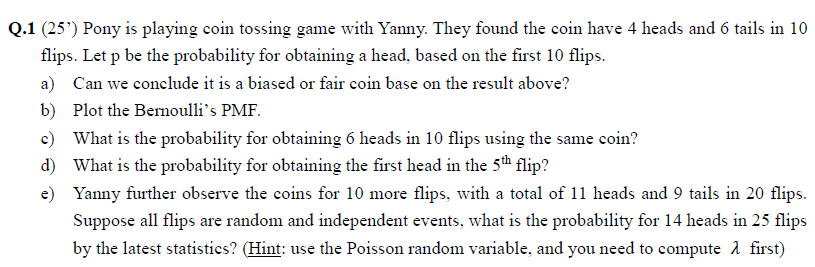Q.1 (25') Pony is playing coin tossing game with Yanny. They found the coin have 4 heads and 6 tails in 10 flips. Let p be the probability for obtaining a head, based on the first 10 flips a) Can we conclude it is a biased or fair coin base on the result above? b) Plot the Bernoulli's PMF What is the probability for obtaining 6 heads in 10 flips using the same coin? d) What is the probability for...

• ### Question Completion Status: QUESTION 1 Use the at least once rule to find the indicated probability....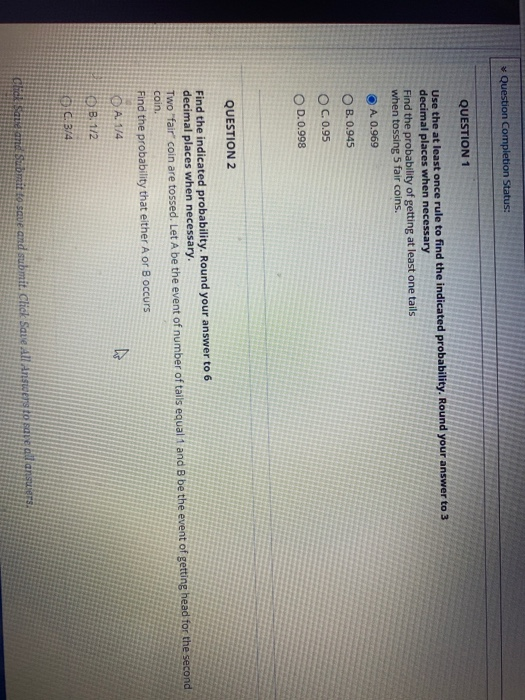Question Completion Status: QUESTION 1 Use the at least once rule to find the indicated probability. Round your answer to 3 decimal places when necessary Find the probability of getting at least one tails when tossing 5 fair coins. O A 0.969 OB. 0.945 OC 0.95 OD. 0.998 QUESTION 2 Find the indicated probability. Round your answer to 6 decimal places when necessary. Two "fair" coin are tossed. Let A be the event of number of tails equal 1 and...

• ### 1.) Determine whether the following individual events are overlapping or​ non-overlapping. Then find the probability of...

1.) Determine whether the following individual events are overlapping or​ non-overlapping. Then find the probability of the combined event. -Getting a sum of either 2 or 5 on a roll of two dice 2.) Use the​ "at least​ once" rule to find the probabilities of the following event. Getting at least one head when tossing four fair coins. (What is the probability) 3) Determine the probability of having 1 girl and 3 boys in a 4​-child family assuming boys and...

• ### 3. (PMF – 8 points) Consider a sequence of independent trials of fair coin tossing. Let...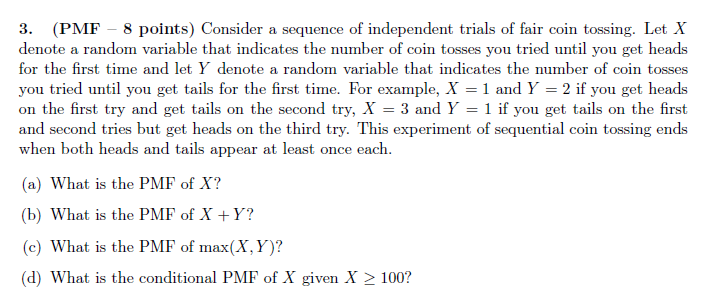3. (PMF – 8 points) Consider a sequence of independent trials of fair coin tossing. Let X denote a random variable that indicates the number of coin tosses you tried until you get heads for the first time and let y denote a random variable that indicates the number of coin tosses you tried until you get tails for the first time. For example, X = 1 and Y = 2 if you get heads on the first try and...

• ### 4. Let 210,1,2) be the outcome space in a model for tossing a coin twice and...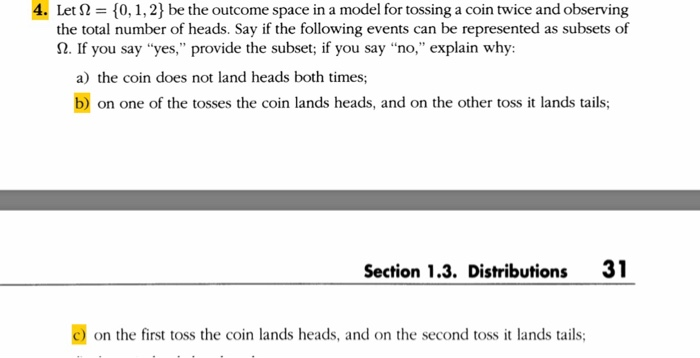4. Let 210,1,2) be the outcome space in a model for tossing a coin twice and observing the total number of heads. Say if the following events can be represented as subsets of Ω. If you say "yes," provide the subset; if you say "no," explain why: a) the coin does not land heads both times; bon one of the tosses the coin lands heads, and on the other toss it lands tails; Section 1.3. Distributions 31 C) on the...

• ### Problem 2: Tails and (Heads or Tails?) Alice and Bob play a coin-tossing game. A fair...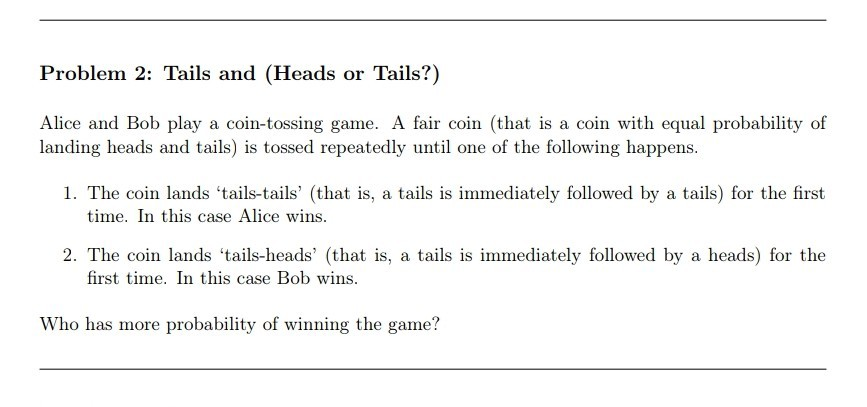Problem 2: Tails and (Heads or Tails?) Alice and Bob play a coin-tossing game. A fair coin (that is a coin with equal probability of 1. The coin lands 'tails-tails' (that is, a tails is immediately followed by a tails) for the first 2. The coin lands 'tails-heads (that is, a tails is immediately followed by a heads) for the landing heads and tails) is tossed repeatedly until one of the following happens time. In this case Alice wins. first...

• ### 3.1 An experiment consists of tossing a fair coin 5 times. (a) Find the probability mass...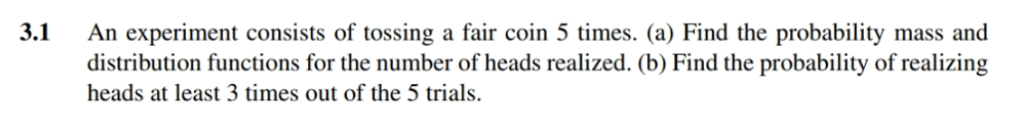3.1 An experiment consists of tossing a fair coin 5 times. (a) Find the probability mass and distribution functions for the number of heads realized. (b) Find the probability of realizing heads at least 3 times out of the 5 trials.

• ### Using c++ Write a function named coinToss that simulates tossing a coin. When you call the...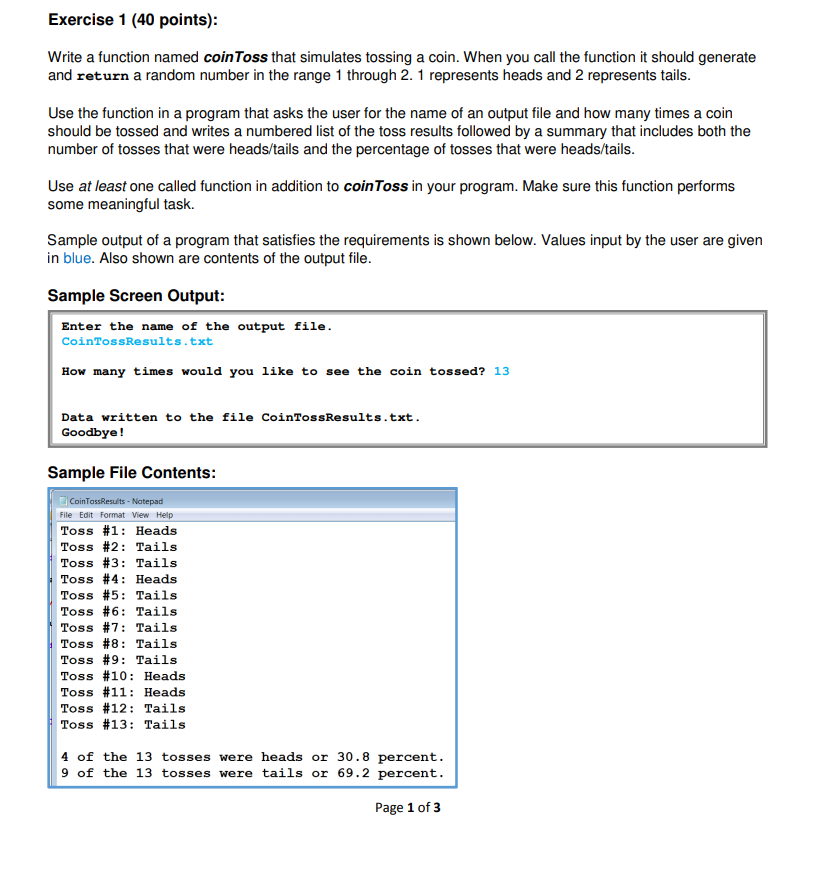Using c++ Write a function named coinToss that simulates tossing a coin. When you call the function it should generate and return a random number in the range 1 through 2. 1 represents heads and 2 represents tails. Exercise 1 (40 points) Write a function named coinToss that simulates tossing a coin. When you call the function it should generate and return a random number in the range 1 through 2.1 represents heads and 2 represents tails Use the function...

• ### Complete the following method. /** * This method calculates the probability of tossing a * coin...

Complete the following method. /** * This method calculates the probability of tossing a * coin n times and and getting n heads. * * The probability of getting a single head is 0.5. The * probability of getting two heads is 0.5*0.5. * The probability of n heads is 0.5*0.5*...*0.5 (where there are * n terms in the product). * * @param n The number of tosses * @return The probability of n heads in n tosses */ public...

• ### Assume that a coin is flipped where the probability of coin lands "Heads" is 0.49. The...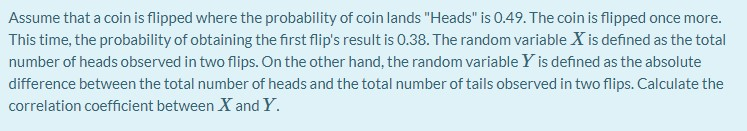Assume that a coin is flipped where the probability of coin lands "Heads" is 0.49. The coin is flipped once more. This time, the probability of obtaining the first flip's result is 0.38. The random variable X is defined as the total number of heads observed in two flips. On the other hand, the random variable Y is defined as the absolute difference between the total number of heads and the total number of tails observed in two flips. Calculate...